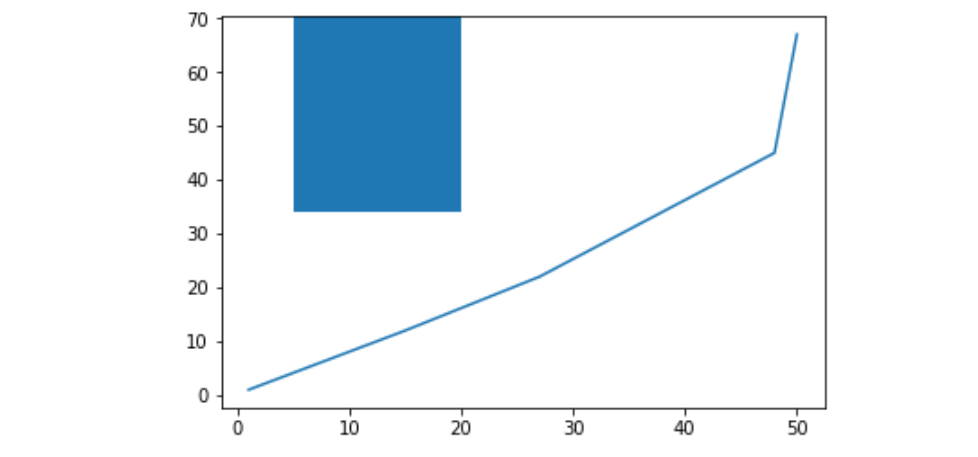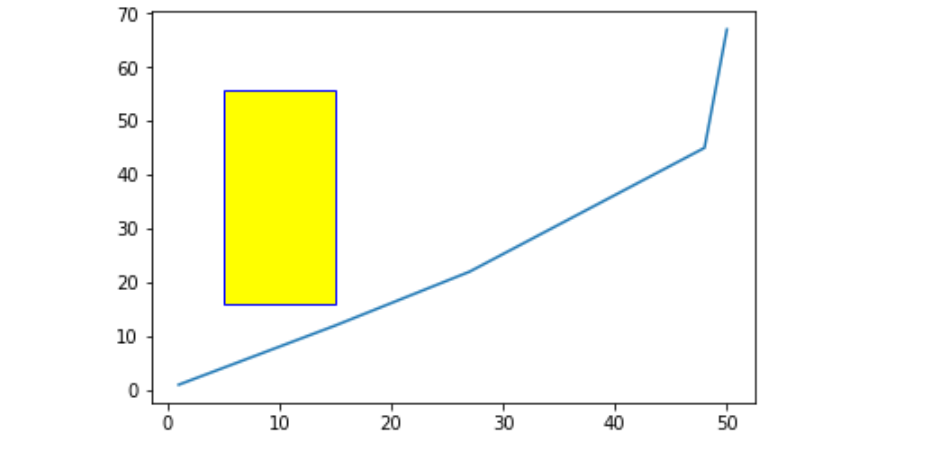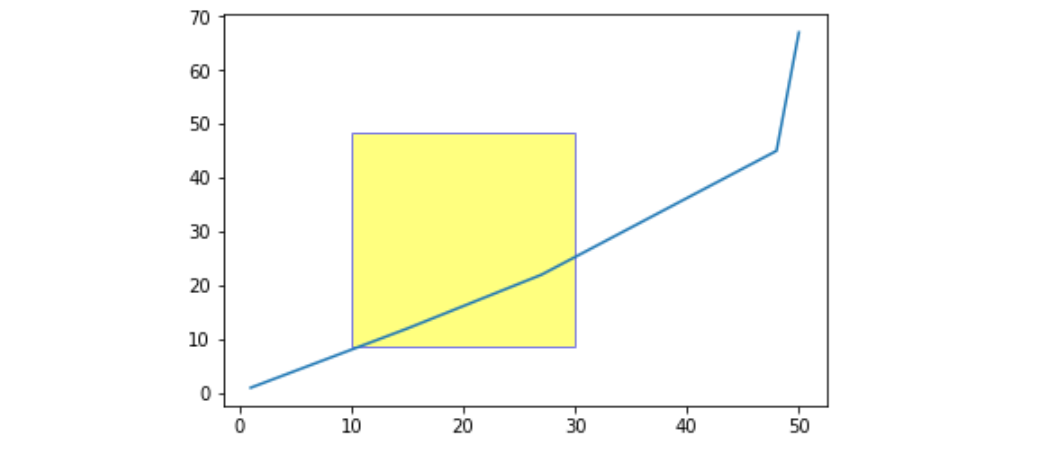# Matplotlib.pyplot.axvspan() in Python

Matplotlib is a plotting library for creating static, animated, and interactive visualizations in Python.\ Pyplot is a Matplotlib module which provides a MATLAB-like interface. Matplotlib is designed to be as usable as MATLAB, with the ability to use Python and the advantage of being free and open-source.

## matplotlib.pyplot.axvspan()

This function sets the vertical rectangle across the axes of the plot

Syntax: matplotlib.pyplot.axvspan(xmin, xmax, ymin=0, ymax=1, **kwargs)

Parameters:
xmin :Number indicating the starting position of the vertical rectangle on X-axis
xmin :Number indicating the ending position of the vertical rectangle on X-axis
ymin :vertical rectangle starting position on y axis, it will take values between 0 and 1, 0 being bottom of the axis, 1 being top of the axis
ymax :vertical rectangle ending position on y axis, it will take values between 0 and 1, 0 being bottom of the axis, 1 being top of the axis
**kwargs :Other optional parameters to change the properties of the rectangle, like changing color etc.

Example #1:

 `# Importing matplotlib.pyplot as plt ` `import` `matplotlib.pyplot as plt ` ` `  `# Initializing x and y ` `x ``=``[``1``, ``15``, ``27``, ``48``, ``50``] ` `y ``=``[``1``, ``12``, ``22``, ``45``, ``67``] ` ` `  `# Plotting the graph ` `plt.plot(x, y) ` ` `  `# Drawing rectangle starting  ` `# x = 5 and extending till x = 20 ` `# With vertical span starting at  ` `# half the length of y-axis(ymin = 0.5) ` `# And extending till the top of  ` `# axis(ymax = 1) ` `plt.axvspan(``5``, ``20``, ymin ``=` `0.5``, ymax ``=` `1``) ` `plt.show() `

Output:Example #2:

 `import` `matplotlib.pyplot as plt ` ` `  ` `  `x ``=``[``1``, ``15``, ``27``, ``48``, ``50``] ` `y ``=``[``1``, ``12``, ``22``, ``45``, ``67``] ` ` `  `plt.plot(x, y) ` ` `  `# Drawing rectangle starting  ` `# x = 5 and extending till x = 15 ` `# With vertical span starting at ` `# 25 % the length of y-axis ` `# And extending till the 80 % of ` `# axis And also we are setting  ` `# the color of rectangle to yellow  ` `# and its edge color to blue ` `plt.axvspan(``5``, ``15``, ymin ``=` `0.25``, ` `            ``ymax ``=` `0.80``, ec ``=``'blue'``,  ` `            ``color ``=``'yellow'``) ` ` `  `plt.show() `

Output :Example #3:

 `import` `matplotlib.pyplot as plt ` ` `  ` `  `x ``=``[``1``, ``15``, ``27``, ``48``, ``50``] ` `y ``=``[``1``, ``12``, ``22``, ``45``, ``67``] ` ` `  `plt.plot(x, y) ` ` `  `# Setting alpha will make  ` `# the rectangle transparent ` `plt.axvspan(``10``, ``30``, ymin ``=` `0.15``,  ` `            ``ymax ``=` `0.70``, ec ``=``'blue'``, ` `            ``color ``=``'yellow'``, ` `            ``alpha ``=` `0.5``) ` ` `  `plt.show() `

Output :My Personal Notes arrow_drop_upCheck out this Author's contributed articles.

If you like GeeksforGeeks and would like to contribute, you can also write an article using contribute.geeksforgeeks.org or mail your article to contribute@geeksforgeeks.org. See your article appearing on the GeeksforGeeks main page and help other Geeks.

Please Improve this article if you find anything incorrect by clicking on the "Improve Article" button below.

Article Tags :

Be the First to upvote.

Please write to us at contribute@geeksforgeeks.org to report any issue with the above content.